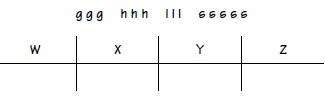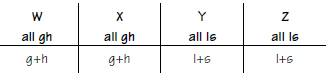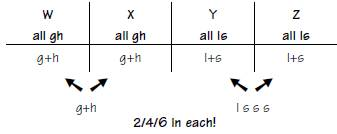Bodhee Prep-Online CAT Coaching | Online CAT Preparation | CAT Online Courses

Get 30% OFF on CAT Crash Course. Code: LASTLAP. Course starts from 18th Sept Enroll Now
| Best Online CAT PreparationFor Enquiry CALL @ +91-95189-40261

# LRDI Practice Set 21

Petworld has exactly fourteen animals (three gerbils, three hamsters, three lizards, five snakes) that are kept in four separate cages (W, X, Y, Z) according to the following conditions:

1. Each cage contains exactly two, four, or six animals.
2. Any cage containing a gerbil also contains at least one hamster; any cage containing a hamster also contains at least one gerbil.
3. Any cage containing a lizard also contains at least one snake; any cage containing a snake also contains at least one lizard.
4. Neither cage Y nor cage Z contains a gerbil.
5. Neither cage W nor cage X contains a lizard.
1. Which one of the following could be a complete and accurate list of the animals kept in cages W and Y?
1. W: one gerbil and one hamster

Y: two lizards and two snakes

1. W: one gerbil and two hamsters

Y: one lizard and three snakes

1. W: two gerbils and two hamsters

Y: one lizard and four snakes

1. W: two gerbils and two hamsters

Y: three lizards and one snake

1. W: two gerbils and two lizards

Y: two hamsters and two snakes

1. If there are exactly two hamsters in cage W and the number of gerbils in cage X is equal to the number of snakes in cage Y, then the number of snakes in cage Z must be exactly
1. one
2. two
3. three
4. four
5. five
1. If cage Z contains exactly twice as many lizards as cage Y, which one of the following can be true?
1. Cage Y contains exactly two lizards.
2. Cage Y contains exactly two snakes.
3. Cage Y contains exactly four animals.
4. Cage Z contains exactly three snakes.
5. Cage Z contains exactly two animals.
1. If the number of animals in cage W is equal to the number of animals in cage Z, then which one of the following can be true?
1. Cage W contains exactly six animals.
2. Cage X contains exactly six animals.
3. Cage Y contains exactly one snake.
4. Cage Y contains exactly three snakes.
5. Cage Z contains exactly four snakes.
1. If cage Y contains six animals, which one of the following must be true?
1. Cage W contains two gerbils.
2. Cage X contains four animals.
3. Cage Z contains two snakes.
4. The number of snakes in cage Y is equal to the number of lizards in cage Y.
5. The number of snakes in cage Z is equal to the number of lizards in cage Z.
1. At most, how many snakes can occupy cage Y at any one time?
1. one
2. two
3. three
4. four
5. five

The Action: Immediately upon seeing “14 animals. . . in four separate cages,” you have to figure that your job will be to place the former in the latter—to carry out, in short, a grouping set of distribution. And so it turns out. We don’t at first know the impact of the information about the different types and numbers of animals, but your hunch might’ve been that in some way it impacts the placement. And that hunch turns out to be correct too. The Key Issues will be:

1) How many animals must or can be in each cage?

2) Which animals must, could, or cannot be caged with each other?

Because of the unusually large number of entities (14) and the odd number breakdown of animal types (3/3/3/5), you might have wanted to save this set till later in the 35 minutes. Set 3, and especially Set 2, might have struck you as more appealing in terms of starting off the section.

The Initial Setup: You’ll likely want to picture the four cages as small boxes or circles, and label them, and make a roster of the entities. Since the cages are capitalized already, perhaps using lower-case letters for the 14 animals makes sense.The Rules:

1) Rule 1 is best left till last. It’s too vague. “Two, four, or six per cage”? Let’s pass over that until we can make it more concrete.

2) This rule’s two clauses make it clear that wherever you find a gerbil (g) you will find at least one hamster (h), and vice versa. So right away, we see that g’s and h’s are linked.

Consider the contrapositives here: If a cage lacks a hamster, then it cannot contain a gerbil. And if it lacks a gerbil, then it cannot contain a hamster. This cements the link even more.

3) gives us a similar link for lizards and snakes. It works, logically speaking, exactly as Rule 2 works. The presence of a lizard requires the presence of a snake, and vice versa; and if a cage lacks one, it must lack the other.

4) Finally, some concreteness. If neither Y nor Z contains a gerbil—remember to turn negatives into positives—then all the gerbils must go into cages W and X. You can note that under your drawn cages W and X: “All g’s.”

5) Same as Rule 4, different terms. Since cages W and X fail to contain lizards, all the lizards will be found in cages Y and Z. So note that in your sketch of those cages: “All l’s.”

Key Deductions: Rule 4 links up with Rule 2, and Rule 5 with Rule 3. Let’s take them step by step and in turn.

There are no gerbils in cages Y and Z, but without a gerbil in a cage we cannot place a hamster there. Conclusion?: There can be no hamsters in cages Y and Z either. Therefore all of the hamsters and gerbils will be placed in cages W and X.

Same deal with Rules 5 and 3. Cages W and X lack lizards, which means (Rule 3) those cages must lack snakes as well. Hence all the lizards and snakes will occupy cages Y and Z. Now, finally, Rule 1 can help us. No cage is empty, and the minimum number of creatures per cage is two. Well, given the interrelationships of the hamster/gerbil and lizard/snake pairs, we can include this much as minimum information:What’s left? There’s one gerbil and one hamster left for cages W and X. They can’t be split between those cages (that’d make for 3 creatures per cage, a violation of Rule 1). So one of the cages will get the remaining g+h pair. The outcome is very neat: Of cages W and X, one will get just g+h, the other will get gghh. Things are slightly less neat for cages Y and Z. Four creatures (one lizard and three snakes; see above sketch) remain. They will have to be placed in cages Y and Z. But how? And in what numbers? Each cage contains its required one snake/one lizard minimum—all that’s prohibited is that a cage contain snakes only or lizards only. So those four remaining creatures can be placed in either cage Y or Z, as long as Rule 1’s number rules are followed. To be precise, either Y and Z will get 4 creatures apiece, or one will get 2 and the other 6.

The Final Visualization:The Questions:

1. (A)

As long as you consulted your sketches carefully and used the Kaplan method for this acceptability question, you should have been OK. (E) directly violates Rule 5 by giving lizards to cage W. Check the arithmetic (Rule 1): (B) is wrong to give a cage three animals, and (C) is wrong to give a cage five. Finally, look at the distribution: If cage Y gets three lizards (D), then it gets all the lizards, and that leaves cage Z with none. Can’t be: (D) would make it impossible to form a valid cage Z. (A) is correct by default.

2. (D)

The first if-clause is easier to deal with: If cage W gets two hamsters, then it must get two gerbils as well (to avoid violating Rule 1). That leaves a single gerbil and hamster for cage X—which, the stem goes on to tell us, has the same number of gerbils as cage Y has snakes. One! Cage Y gets a single snake, which means that all of the remaining snakes go into cage Z; five minus one is four, choice (D).

3. (C)

The arithmetic here may be even easier than that of Q. 2. There are only three lizards to begin with, so the if-clause here must mean that Y has one lizard and Z two. (Note that that makes (A) wrong automatically.) The five snakes can be divvied up among cages Y and Z in several ways, as long as each cage contains at least one snake and an overall total of two, four, or six animals. (C) works fine: The two cages could indeed have four animals each— LSSS in Y, and LLSS in Z—so (C) is the statement that “can be true.” (B) Exactly two snakes + Y’s one lizard would result in three animals in cage Y, a violation of Rule 1. (D) has the same problem as (B): If we add exactly three snakes to the two lizards that we determined must be in Z (in order to satisfy the stem), we end up with an odd number of critters in both Y and Z, in violation of Rule 1. (E) Given the stem, Z has to have two lizards to Y’s one, and Z needs snakes beyond that. So (E) is impossible.

4. (D)

Looking at the master sketch, if W and Z are to get the same number of animals, that number must be two or four—and if you sketch the two possibilities out briefly, it may make the work go quicker. If W and Z each get two, then each of them gets its minimum allotment of a gerbil and hamster for W and a lizard and snake for Z, leaving X and Y with all of the remains. If W and Z each get four, then W gets two gerbils and two hamsters, while Z gets some combination of lizards and snakes equaling four. Your best bet is to check each choice against these possible arrangements: (A), (B) A glance at our master sketch reveals that there are only six animals total (3g, 3h) to be split between cages W and X. If either cage got all six, then the other would be left with zero, in violation of Rule 1. (C) In option 1 above, Y gets four snakes, and in option 2, Y gets at least two snakes. Exactly one snake for Y is therefore not an option. (D) works: Y could get a lizard and three snakes, with Z getting two of each (and W getting its four animals). On test day you need look no further, but for the record: (E) In option 1, Z has just one snake, and in option 2, Z gets at most three snakes.

5. (E)

Cage Y’s six animals would have to include the LS pair that’s always there, and the four remaining animals, giving us LLSSSS in Y, and LS in Z. (E) is therefore correct—exactly one of each type is present in Z. (D) is flat wrong, and so is (C): Z gets only one snake. All of this distribution, of course, has no impact on cages W and X. In point of fact either (A) or (B) has to be true and the other false, but we can’t tell which is which, and the Y/Z situation doesn’t help any.

6. (D)

As we just saw in the previous question, Y can contain four snakes and two lizards, leaving one snake and one lizard for Z. The maximum number of snakes for Y is 4, choice (D).

Online CAT LRDI Course @ INR 3999 only

#### CAT 2023 Mock Test Series

• 400+ Topic Tests
• 45 Sectional tests
• 20 Mock Tests (Video Solutions)
• Only at INR 2499

20% Discount Code: GET20

FREE CAT Prep Whatsapp Group

CAT 2023 Online Course at affordable price